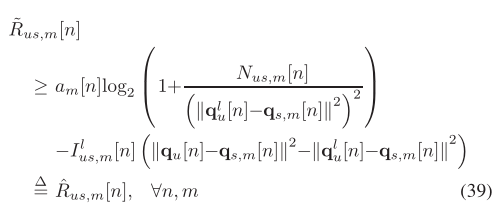# How to express matrix initial constraint in cvx

i’m working on an UAV trajedy optimization problem,and my code is like following

``````cvx_begin
%two-dimension location
variable Q(2,100)
%objective
expressions R(100)
for i = 1:100
R(i) = pow_pos(norm(Q(:,i)-W(:,i),2),2);
end
minimize sum(R)
subject to
%initial location constraint
Q(:,1) == [0;0];
Q(:,100) == [3000;0];
for i = 2:100
norm(Q(:,i)-Q(:,i-1),2) <= 40;
end
cvx_end
``````

it doesn’t work,the status is ‘‘infeasiable’’,but if I delete the initial location constraint ,it works

``````cvx_begin
%two-dimension location
variable Q(2,100)
%objective
expressions R(100)
for i = 1:100
R(i) = pow_pos(norm(Q(:,i)-W(:,i),2),2);
end
minimize sum(R)
subject to
%initial location constraint
for i = 2:100
norm(Q(:,i)-Q(:,i-1),2) <= 40;
end
cvx_end
``````

so how can i solve it?I would be grateful if you could answer my questions. Thanks, everyone.

All but section 1 of https://yalmip.github.io/debugginginfeasible also applies to CVX.

thank you for your help!this is my original problem formula,and I simplify it to this,

``````cvx_begin
%two-dimension location
variable Q(2,100)
%objective
expressions R(100)
for i = 1:100
R(i) = pow_pos(norm(Q(:,i)-W(:,i),2),2);
end
minimize sum(R)
subject to
%initial location constraint
Q(:,1) == [0;0];
Q(:,100) == [3000;0];
%distance constraint
for i = 2:100
norm(Q(:,i)-Q(:,i-1),2) <= 40;
end
cvx_end
``````

this seems to be convex,but when i run it, the output is Inf and Q is NaN,is that any question with my
object function? I’d really appreciate it if you could help me

Does CVX report it is infeasible? If so, follow the guidance in the link I provided.

yes it is, but i’m very confusing because norm is convex to Q, and square is convex,so the problem seems to be convex, and it should get a solution,and the site you provided is a little bit abstract to meThe problem is convex and the code was accepted by CVX. CVX and the solver it calls concluded it is infeasible. Follow the guidance in the link.

thank you for your help, but it dosen’t work to me because i’m new with itThe problem is actually feasible.

Apply item 2 in the link, which is the first one applicable to CVX. Remove the objective function and see whether it i feasible. You can easily see anlaytically it is feasible. But I ran it in CVX nevertheless, using Mosek as solver.

`````` cvx_begin
%two-dimension location
variable Q(2,100)
%initial location constraint
Q(:,1) == [0;0];
Q(:,100) == [3000;0];
%distance constraint
for i = 2:100
norm(Q(:,i)-Q(:,i-1),2) <= 40;
end
cvx_end
``````

it is reported feasible. I can 't reproduce your problem with the objective function, because you have not provided `W`. Nor have you shown the solver output. Perhaps `W` is badly scaled numerically, and has some non-zero elements which are not within a small number of orders of magnitude.of one, which if so, can confuse the solver.

i’m sorry that i didn’t put the W,here is the W matrix

``````W = ones(2,100);
W(1,:) = linspace(10000,11000,100);
W(2,:) = linspace(1000,1000,100);
cvx_begin
%two-dimension location
variable Q(2,100)
%objective
expressions R(100)
for i = 1:100
R(i) = pow_pos(norm(Q(:,i)-W(:,i),2),2);
end
minimize sum(R)
subject to
%initial location constraint
Q(:,1) == [0;0];
Q(:,100) == [3000;0];
%distance constraint
for i = 2:100
norm(Q(:,i)-Q(:,i-1),2) <= 40;
end
cvx_end
``````

it is because i set the scene as off-shore seas,so the distance is very far.
and the output is here

``````Calling SDPT3 4.0: 1196 variables, 501 equality constraints
------------------------------------------------------------

num. of constraints = 501
dim. of sdp    var  = 200,   num. of sdp  blk  = 100
dim. of socp   var  = 597,   num. of socp blk  = 199
dim. of linear var  = 299
*******************************************************************
SDPT3: Infeasible path-following algorithms
*******************************************************************
version  predcorr  gam  expon  scale_data
HKM      1      0.000   1        0
it pstep dstep pinfeas dinfeas  gap      prim-obj      dual-obj    cputime
-------------------------------------------------------------------
0|0.000|0.000|3.1e+02|3.0e+01|9.4e+08| 2.000200e+06  0.000000e+00| 0:0:00| spchol  1  1
1|0.350|0.630|2.0e+02|1.1e+01|1.0e+09| 4.455869e+06  4.473167e+05| 0:0:00| spchol  1  1
2|0.377|0.925|1.3e+02|1.1e+00|6.4e+08| 2.329032e+07  3.678666e+05| 0:0:00| spchol  1  1
3|0.792|1.000|2.6e+01|1.2e-01|1.6e+08| 2.937066e+07  4.611075e+05| 0:0:00| spchol  1  1
4|0.721|1.000|7.3e+00|6.2e-02|5.3e+07| 1.036118e+07  1.076930e+06| 0:0:00| spchol  1  1
5|0.386|0.286|4.5e+00|5.4e-02|3.9e+07| 9.603712e+06  2.235950e+06| 0:0:00| spchol  1  1
6|0.285|0.235|3.2e+00|4.5e-02|3.2e+07| 9.033413e+06  4.217707e+06| 0:0:00| spchol  1  1
7|0.225|0.105|2.5e+00|4.1e-02|2.7e+07| 9.244942e+06  6.174619e+06| 0:0:00| spchol  1  1
8|0.241|0.126|1.9e+00|3.6e-02|2.3e+07| 9.859460e+06  9.701756e+06| 0:0:00| spchol  1  1
9|0.234|0.136|1.4e+00|3.1e-02|2.0e+07| 1.150227e+07  1.612385e+07| 0:0:00| spchol  1  1
10|0.188|0.082|1.2e+00|2.9e-02|1.9e+07| 1.473714e+07  2.380427e+07| 0:0:00| spchol  1  1
11|0.166|0.087|9.7e-01|2.6e-02|1.9e+07| 1.871685e+07  3.447816e+07| 0:0:00| spchol  1  1
12|0.142|0.074|8.4e-01|2.5e-02|2.0e+07| 2.390158e+07  4.648149e+07| 0:0:00| spchol  1  1
13|0.133|0.070|7.2e-01|2.3e-02|2.2e+07| 3.070433e+07  6.148222e+07| 0:0:00| spchol  1  1
14|0.117|0.065|6.4e-01|2.1e-02|2.6e+07| 3.886766e+07  7.976818e+07| 0:0:00| spchol  1  1
15|0.110|0.063|5.7e-01|2.0e-02|3.1e+07| 4.882038e+07  1.008943e+08| 0:0:00| spchol  1  1
16|0.103|0.061|5.1e-01|1.9e-02|3.7e+07| 6.063799e+07  1.256300e+08| 0:0:00| spchol  1  1
17|0.099|0.060|4.6e-01|1.8e-02|4.5e+07| 7.474178e+07  1.546500e+08| 0:0:00| spchol  1  1
18|0.091|0.058|4.2e-01|1.7e-02|5.4e+07| 9.058117e+07  1.878231e+08| 0:0:00| spchol  1  1
19|0.088|0.059|3.8e-01|1.6e-02|6.5e+07| 1.091171e+08  2.272778e+08| 0:0:00| spchol  1  1
20|0.087|0.059|3.5e-01|1.5e-02|7.9e+07| 1.311388e+08  2.725813e+08| 0:0:00| spchol  1  1
21|0.102|0.059|3.1e-01|1.4e-02|9.5e+07| 1.617328e+08  3.244172e+08| 0:0:00| spchol  1  1
22|0.115|0.054|2.8e-01|1.3e-02|1.1e+08| 2.017888e+08  3.802277e+08| 0:0:00| spchol  1  1
23|0.142|0.057|2.4e-01|1.2e-02|1.3e+08| 2.587567e+08  4.494511e+08| 0:0:00| spchol  1  1
24|0.112|0.050|2.1e-01|1.2e-02|1.6e+08| 3.121029e+08  5.242561e+08| 0:0:00| spchol  1  1
25|0.068|0.093|2.0e-01|1.1e-02|1.9e+08| 3.505001e+08  6.719893e+08| 0:0:00| spchol  1  1
26|0.081|0.128|1.8e-01|9.3e-03|2.4e+08| 4.094216e+08  8.698204e+08| 0:0:00| spchol  1  1
27|0.077|0.118|1.7e-01|8.2e-03|3.0e+08| 4.789307e+08  1.039169e+09| 0:0:00| spchol  1  1
28|0.096|0.184|1.5e-01|6.7e-03|4.0e+08| 5.780055e+08  1.288876e+09| 0:0:00| spchol  1  1
29|0.047|0.438|1.4e-01|3.8e-03|6.8e+08| 6.357415e+08  1.784513e+09| 0:0:00| spchol  1  1
30|0.039|0.738|1.4e-01|9.9e-04|1.0e+09| 7.153826e+08  1.827097e+09| 0:0:00| spchol  1  1
31|0.092|0.254|1.3e-01|7.4e-04|1.3e+09| 8.830092e+08  2.316191e+09| 0:0:00| spchol  1  1
32|0.173|0.228|1.0e-01|5.7e-04|1.4e+09| 1.326131e+09  2.408991e+09| 0:0:00| spchol  1  1
33|0.140|0.721|8.9e-02|1.6e-04|1.8e+09| 1.594810e+09  2.937641e+09| 0:0:00| spchol  1  1
34|0.264|0.786|6.6e-02|1.8e-02|1.8e+09| 2.165306e+09  3.227323e+09| 0:0:00|
sqlp stop: primal problem is suspected of being infeasible
-------------------------------------------------------------------
number of iterations   = 34
residual of primal infeasibility
certificate (y,Z)      = 3.12e-09
reldist to infeas.    <= 5.12e-09
Total CPU time (secs)  = 0.30
CPU time per iteration = 0.01
termination code       =  1
DIMACS: 8.5e-02  0.0e+00  9.8e-02  0.0e+00  -2.0e-01  3.3e-01
-------------------------------------------------------------------

------------------------------------------------------------
Status: Infeasible
Optimal value (cvx_optval): +Inf
``````

it is the bad scaling in `W` causing the difficulty, which is exacerbated by the squaring going on in the objective function.

Divide `W` and the RHS of all constraints by 1000. Which would change meters to km. Then the problem is easily solved by SDPT3 and Mosek, with cvx_optval = 7513.83. You need to multiply this cvx_optval by 1000^2 to put it back in original units. And multiply the optimal `Q` by 1000 to put it back in the original units.